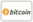Bookcover of Maxwell's equations in curved spacetime
Booktitle:

# Maxwell's equations in curved spacetime

Alphascript Publishing (2010-11-28 )eligible for voucher
ISBN-13:

### 978-613-0-21307-7

ISBN-10:
6130213077
EAN:
9786130213077
Book language:
English
Blurb/Shorttext:
Please note that the content of this book primarily consists of articles available from Wikipedia or other free sources online.In physics, Maxwell's equations in curved spacetime govern the dynamics of the electromagnetic field in curved spacetime (where the metric may not be the Minkowski metric) or where one uses an arbitrary (not necessarily Cartesian) coordinate system. These equations can be viewed as a generalization of the vacuum Maxwell's equations which are normally formulated in the local coordinates of flat spacetime. But because general relativity dictates that the presence of electromagnetic fields (or energy/matter in general) induce curvature in spacetime, Maxwell's equations in flat spacetime should be viewed as a convenient approximation.However, this formulation of Maxwell equations is only useful for the vacuum Maxwell equations, also called the "microscopic" Maxwell equations. For the "macroscopic" Maxwell equations in matter, the presence of materials establishes a reference frame and the equations are no longer covariant. Maxwell equations for the macroscopic electromagnetic field in matter may be deduced from postulated Maxwell equations for the microscopic electromagnetic field.
Publishing house:
Alphascript Publishing
Website:
https://www.alphascript-publishing.com/
Edited by:
Frederic P. Miller, Agnes F. Vandome, John McBrewster
Number of pages:
108
Published on:
2010-11-28
Stock:
Available
Category:
Psychology
Price:
39.00 €
Keywords:
Local, Tensor, Relativity, Classical, space### Categories